• 学号姓名班级实验地点实验日期课程名称实验课时实验名称动态规划求解背包问题实验目的通过上机实验，要求掌握动态规划算法的问题描述、算法设计思想、程序设计。实验环境Pycharm实验内容和原理动态规划通常是用来...
学号姓名班级实验地点实验日期课程名称实验课时实验名称动态规划法求解背包问题实验目的通过上机实验，要求掌握动态规划算法的问题描述、算法设计思想、程序设计。实验环境Pycharm实验内容和原理动态规划通常是用来求解最优化问题.这类问题可以有很多个可行解，每个解都有一个值，我们希望寻找最优值(最大值或者最小值)的解。我们称这样的解为问题的一个最优解。因为有可能有多个解都达到最优值，但是在求解原问题的最优解的时候只需要找出其中的一个就可以了。就像矩阵链乘法问题中，选择最佳分割位置k的时候，是只需要判断新的代价是否比之前的选中的k时的代价小，如果不小则不变换k的值。这就说明了，是可能出现另外一个分割位置与当前记录的k的位置的代价是一样的，这就说明了k的最佳划分位置不止一个，即原问题的最优解可能不是唯一的，但是动态规划则是求出原问题的一个最优解。通常按照如下四个步骤来设计一个动态规划算法：1.刻画一个最优解的结构特征；2.递归地定义最优解的值；3.计算最优解的值，通常采用自底向上的方法；4.利用计算出的线性构造一个最优解。
展开全文• 算法设计实验报告，包括：贪心法求解背包问题的基本思想动态规划法求解0/1背包问题的基本思想及各自的时间复杂度分析，两种问题的区别，C++实现代码，运行截图，实验心得。
• 动态规划（DP）初探 DP的思想是：将一个大问题求解转化为一些更小问题求解。我们假设对于一个更小的问题已经有了最优解答，在此基础上我们build up the bigger problems。这种分而治之的思想非常像递归，但是...
Dynamic Programming
动态规划（DP）初探
DP的思想是：将一个大问题的求解转化为一些更小问题的求解。我们假设对于一个更小的问题已经有了最优解答，在此基础上我们build up the bigger problems。这种分而治之的思想非常像递归，但是递归通常会招致不必要的算力消耗，因此 DP使用了 bottom up的方法，有效地节省了算力。
DP guarantees to find the best solution
(Basic principles: 1. Divide and Conquer; 2. Bottom Up computation)
当我们谈起DP，其实可以预设一个很大的 table，我们要做的事情其实就是填表。
Take knapsack as an example
这里还是以 knapsack problem为例，假设问题如下：
给定一个 Capacity为 k的 knapsack，给定一组物品并用 1,2,…,n标记，物品i的价值为$v_i$，重量为 $w_i$. $I = {1,2,…,n }$.
希望找到一个最优选择，使得在 knapsack可以装下的情况下，拿走物品的总价值最大。
我们可以建模如下：
$\max \sum_{i\in I}v_i x_i\\ s.t. \sum_{i\in I}v_i x_i \le k \\ x_i \in \{0,1\}, i\in I$
现在假设 $O(p,m)$是当在 knapsack的 capacity为p，物品下标为 1,2,…,m的时候的最优选择下的总价值。这些就是子问题。
我们所关心的是 $O(k,n)$的值，并找出这个最大值对应的最优选择。
如果我们假设 $O(p,j)$ 已经已知，对于$p\le k, j \le m$，那么，如果我们在选择 $O(p, m+1)$的时候，无非就是选择物品m+1或者不选择它。
如果选择了物品m+1，那么我们还剩下 $p-w_{m+1}$ 的背包容量（如果$p-w_{m+1}$ 是一个负数，显然就不装物品m+1了），以及物品 1,…,m待选择，这种情况下我们最好的选择方法就是按照 $O(p-w_{i+1}, m)$来选择。因此，在选择物品m+1的情况下，能获得的最大价值是 $v_{m+1} + O(p-w_{i+1}, m)$
如果不选择物品 m+1，那么我们就有一个容量p的背包和物品 1,…,m待选择。因此，在不选择物品 m+1的情况下，能获得的最大价值的 $O(p, m)$
因此，我们选择 $O(p,m+1) := \max (v_{m+1} + O(p-w_{i+1}, m),O(p, m) )$，这就完成了从更简单的subproblem到bigger problems的构建。
至于base case，也就是 $O(p, 1), 1\le p \le k$，这个的填充方法不过是：能装下就装并填上 $v_1$，不能装就填0.
Simple as that。所做的不过是 fill in a k times n table.
Recover the best choice
当然，还有一个问题就是：如何从这个 中 recover最优的选择？这个同样是不难的。首先，我们注意到，如果在选择 $O(p, m+1)$的时候，我们不选择物品m+1，那么 $O(p,m+1) = O(p,m)$。因此，只需要检查这个是否相等，我们就可以判断是否最优选择包含了这个物品。
嗯… Something like this: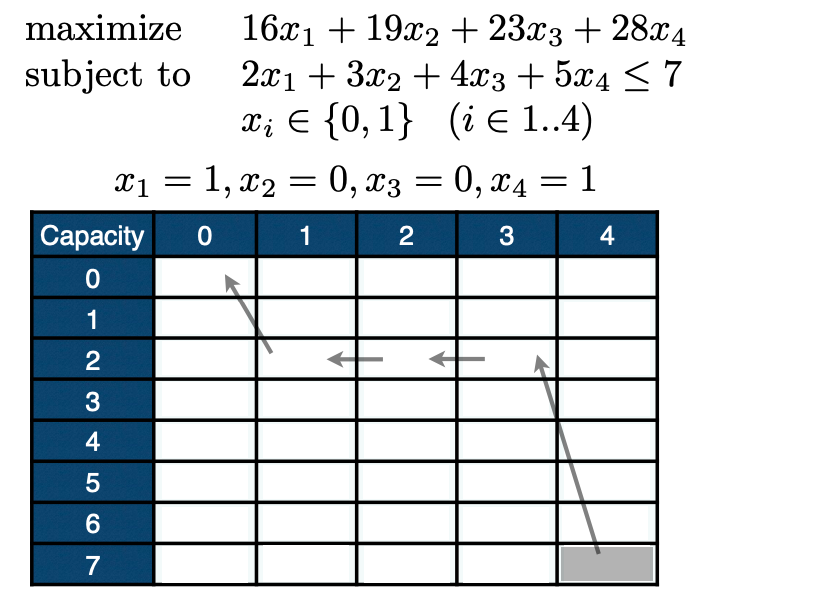如果 O(7,4) > O(7,3)，那么说明物品4是被选择的。然后发现 O(2,3) = O(2,2)，这说明没有选择物品3；然后是O(2,2) = O(2,1)，说明物品2也没有被选择。最后发现 O(2,1) > 0，因此物品1是被选择了的。综上所述，最优选择是 (1,0,0,1)
The Complexity is pseudo-polynomial
Fill in the table 的算法复杂度是 O(kn)。如果我们只关注n，那么这个算法是 linear in n。但是，因为我们仅仅需要 $\log k$ bits 来 represent k，这个复杂度实际上是 $O(2^{\log k} n)$，因此是 exponenetial in terms of the input size
（这个我也有点迷糊… 看了挺多回答也半蒙半懂的。我现在的状态就像是在问“为什么鸽子这么大”… ）
所以当 k is small, DP is quite efficient for knapsack problems
但是如果 k很大，我们可能就得另觅他法了。
对于DP的介绍就先到这里~


展开全文coursera 算法
• 问题描述 假设有一个背包,它的最大承重为Nkg(N是整数),现有k个物品T1, T2, …,Tk,它们的重量分别为G1, G2, …Gk.它们的价格分别为P1, P2, ...动态规划思想是设计一个二元函数dp,它返回的是当前条件下的最优解.然后我
问题描述
假设有一个背包,它的最大承重为Nkg(N是整数),现有k个物品T1, T2, …,Tk,它们的重量分别为G1, G2, …Gk.它们的价格分别为P1, P2, …,Pk.
问如何把这些物品装进背包(不能超过背包的承重),使得背包里的物品价格最高?最高价格是多少?
分析
假设
为了简单理解,我们取N=4,共有三件物品,运动鞋,电饭锅和电风扇.它们的重量分别是1kg, 4kg, 3kg,价值分别是150, 300, 200元.
动态规划的思想是设计一个二元函数dp,它返回的是当前条件下的最优解.然后我们可以设计一个二维数组,根据dp的递推公式逐一地向这个二维数组里填值,当我们填完这个二维数组以后,最后填进去的值就是我们要求的最终的结果.
上面是动态规划算法的一个简单概括,其实它的思想就是把一个大问题分解成若干个小问题,每个小问题我们都得到它的一个最优解,也就是局部最优解,再通过寻找小问题到大问题的递推关系,实现局部最优到整体最优的递进.因此如何找到这个关系,或者说,如何规定这样的二元函数,成了我们解决此问题的关键.
从问题的描述我们可以得知,这个解跟物品的重量,背包的承重,以及物品的价值有关,所以在设计dp数组的时候是否就可以利用到其中两个因素作为这个二元函数的两个自变量呢?因为物品的价值是在前两个变量的确定的前提下才可以确定的,所以取前两个作为dp的自变量,对这个自变量我们需要做点适当的改变,使得它易于计算机理解.
数学化这个问题
我们将各种商品编号,正如问题描述的那样,当我们称呼这个商品时,采用编号代替它原本的名字,比如第i个商品,我们把商品编号作为dp函数的第一个自变量,第二个自变量是背包的承重,我们可以把这个背包的承重从小到大排,即从1kg开始,每次递增1kg,直到达到真正的背包承重(这也就是把问题分解成若干个子问题的思想),可以认为每个承重表示一个背包,但最后一个背包才是我们题目中的背包.
根据这样设计的二维数组如下所示: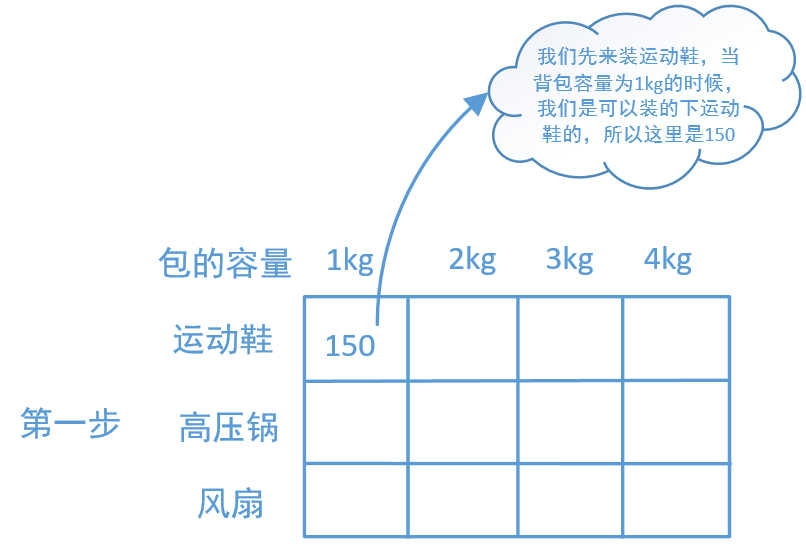这样的话dp[i][j]它表示的就是利用第j个背包来装从第1个商品到第i个商品中的任意商品组合时,它们的最大价值.比如第1行第1列就是说如果包的容量为1kg,商品只有一个运动鞋,那么它显然是可以装到背包里去的(这里我们又发现,其实没有必要将重量从1开始,只要从最轻的那个物品重量开始递增就可以了),所以填的是这个运动鞋的价值150,
因为后面的背包容量比它大,所以显然也能装下,因此后面的列里所填的数字都是150.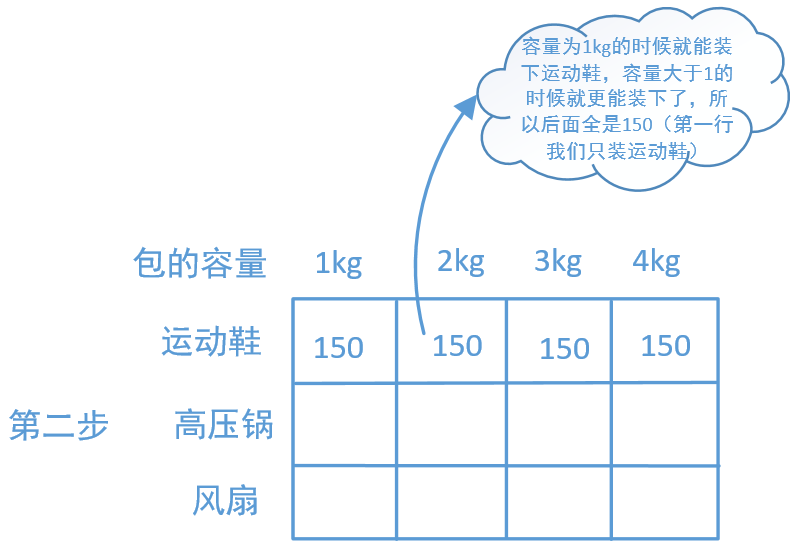假设这些东西的重量和价格都被用以数组表示,weight = {1, 4, 3},value = {150, 300, 200},背包的承重(容量)contain_weight = {1, 2, 3, 4}.显然我们是出于这样的考虑:我们选择的物品的集合S应当满足重量不超过此时的contain_weight[j],也就是$\sum_{t\in S} weight[t]\leqslant contain\_weight[j]$,并且$dp[i][j] = max\{\sum_{t\in S} value[t]\}$.当我们要对dp[i][j]赋值时,我们就要考虑一个包含商品i的集合,这个集合要满足上面两个表达式.如果第一个不满足,也就是这个商品超过了背包的承重,那么无论是什么样的包含这个商品的集合都不可能被装入背包中,此时我们也不能不填,但这个时候所谓的最大的物品价值就是不包含这个商品i的了,此时我们的最大价值就应该是dp[i-1][j],也就是上一行的数据粘贴下来就可以了,这种情况一般不会出现.但也要考虑到.
一般不等式1是满足的,那么就存在这样的物品集合$S_{i}$(它表示这个物品集合一定包含i这个物品),接下来就考虑如何实现价值最大化,在此之前,我们最大化的价值是dp[i-1][j],它对应的物品集合我们不清楚,但一定不包含商品i,我们需要找到这样的物品集合$S_{i}$跟前面dp[i-1][j]对应的物品集合没有什么必然联系,这个商品集合$S_{i}$一定要满足它里面的商品重量不超过contain_weight[j],也就是$\sum_{t\in S_{i}}weight[t]\leqslant contain\_weight[j]$,并且
$\sum_{t\in S_{i}}value[t] = max$,也就是如果还有其他的商品组合$S_{i}'$,它们的价值不会超过$S_{i}$的价值.这是一个比较难想的点.但我们一定要利用到前面的数据,我们试想,如果没有这个商品i的话,最大价值我们是知道的但它不是dp[i-1][j],因为我们要考虑的是加上这个商品以后这个商品集合的总价值上升了,但重量也同时增加了,dp[i-1][j]对应的物品集合跟我们的$S_{i}$没有任何必然联系,所以我们就没办法保证加上这个商品以后不会超过此时背包的承重.所以我们所选择的一定是去掉这个商品i重量的contain_weight,比如是contain_weight[k],因为这样它一定满足$contain\_weight[k] + weight[i] \leqslant contain\_weigt[j]$,也就是dp[i-1][k]对应的集合$S_{i-1}$满足它是在没有商品i的时候背包里所能装的最大价值的商品集合,在加上商品i以后价值进一步增加了,并且也不超过此时的背包容量.我们将它抽象化为$max\sum_{t\in S_{i}} value[t] = dp[i-1][j-weight[i]] + value[i]$
所以按照这个思路,接下来的表格补充如下: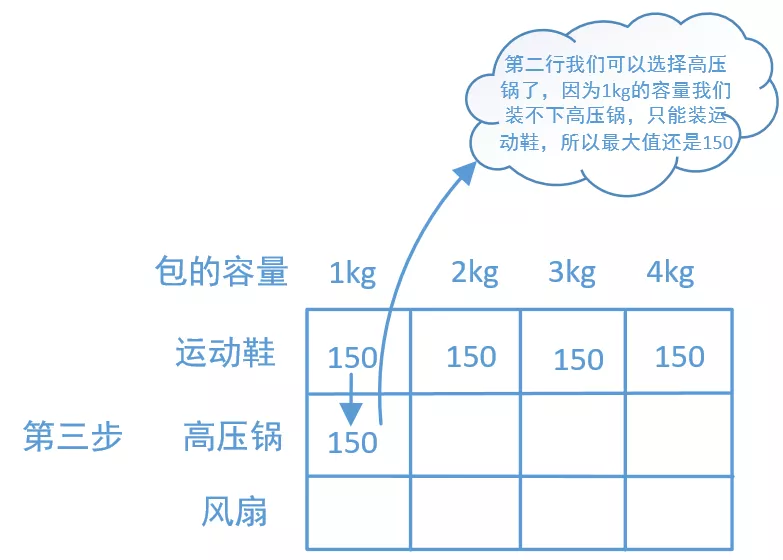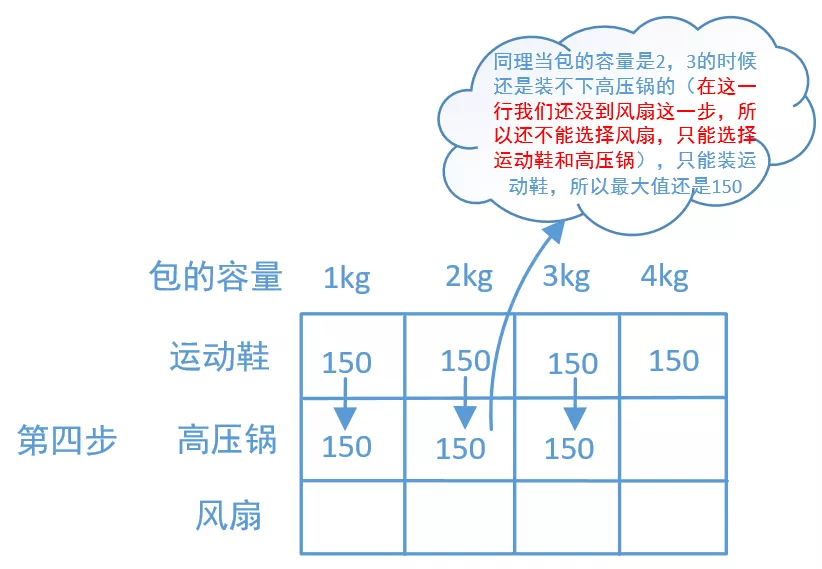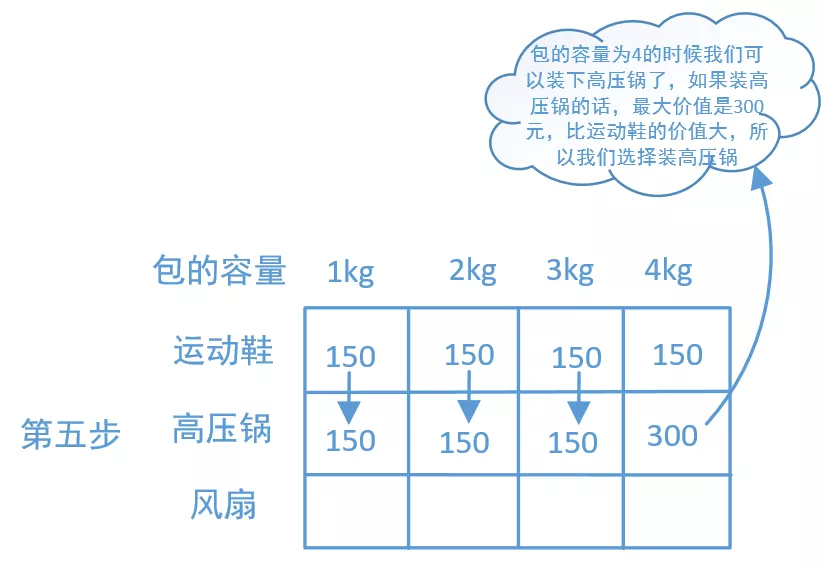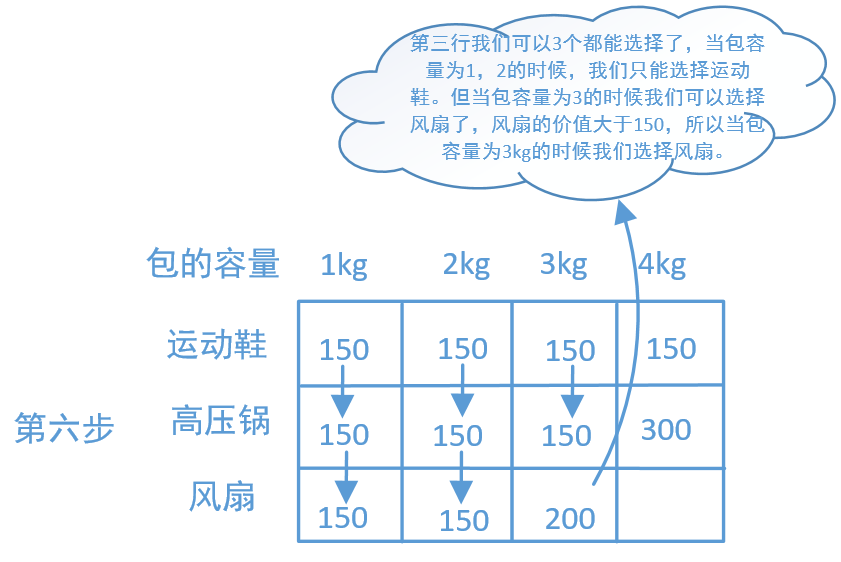最后我们还要比较有这个商品i的集合Si的最大价值和没有这个商品i的集合S’的价值哪个更大,因为我们始终要填入的是最大的价值:
$dp[i][j] = max\{dp[i-1][j], \sum_{S_{i}}value[t]\}$,或者更进一步,
$dp[i][j] = max\{dp[i-1][j], dp[i][j-weight[i]]+value[i]\}$.
这个抉择在我们最后填入dp的时候得到了体现.算法的实现
我们将这个算法的步骤总结如下:
1.设计一个长度为k+1 * N+1 的二维数组(这是为了赋初值和递推),第一行和第一列全置0.分别对value,weight,contain_weight数组初始化.
2.从第一行第一列开始,设计一个两层循环,先遍历列,再遍历行.
3.如果weight[i] > contain_weight[j], dp[i][j] = dp[i-1][j],否则执行4
4.dp[i][j] = max{dp[i-1][j], dp[i-1][j-weight[i]]+value[i]}.
5.循环结束则整个算法结束,最右下角的数字即是最终解.
我们即以前面的假设为例采用python编写此算法.
#取N=4,共有三件物品,它们的重量分别是1kg, 4kg, 3kg,价值分别是150, 300, 200元.
def packagePro():
weight = [-1, 1, 4, 3]#通过增加一个-1使得后面遍历的时候下标跟二维数组的下标一致,下面都是这样处理的
value = [-1, 150, 300, 200]
contain_weight = [-1, 1, 2, 3, 4]
bag_num = 4
good_num = 3
dp = [[0 for j in range(bag_num + 1)] for i in range(good_num + 1)]
for i in range(1, good_num+1):
for j in range(1, bag_num+1):
if weight[i] > contain_weight[j]:
dp[i][j] = dp[i-1][j]
else:
dp[i][j] = max(dp[i-1][j-weight[i]] + value[i], dp[i-1][j])
return dp[good_num][bag_num]

推广到浮点数
其实这个问题不局限于整数,浮点数也是可以的,但是在分解的时候关于背包的承重最好还是用整数,推广到浮点数的时候有一点需要注意,也就是
$dp[i][j] = max\{dp[i-1][j], dp[i][j-weight[i]]+value[i]\}$
这个公式需要做更改,因为这个时候的重量weight[i]不一定是整数,也就是这个时候j-weight[i]不一定是整数,但作为下标一定得是整数,所以这个时候要对j-weight[i]向下取整,即满足条件的集合重量k应是不大于j-weight[i]的最大整数.这个我还没有编,但应该不难,读者感兴趣的话自己编一下.
这里特别感谢这篇文章,所有的插图都来自这里.我只是做了一点自己的补充而已.
https://mp.weixin.qq.com/s/sCLhGsXgGk6ev7wzup0uZQ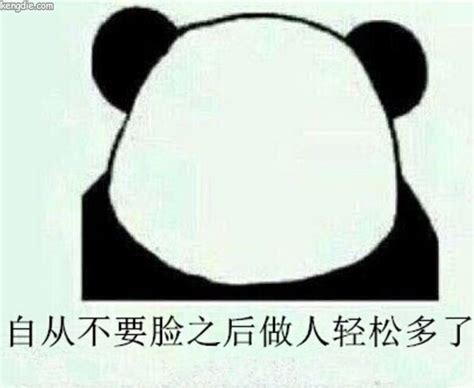展开全文算法 python
• 动态规划思想解决背包问题。过程中减少了不必要的重复计算。
• 继上一篇后续《(29)Go动态规划经典思想-01背包问题》https://www.jianshu.com/p/5c54e32cdd49 问题1：分割等和子集 // 是一个典型的背包问题，从某地方抽取元素，填充某个值 设sum为非空集合所有元素的和，若sum%...
继上一篇后续《(29)Go动态规划经典思想-01背包问题》https://www.jianshu.com/p/5c54e32cdd49
问题1：分割等和子集 //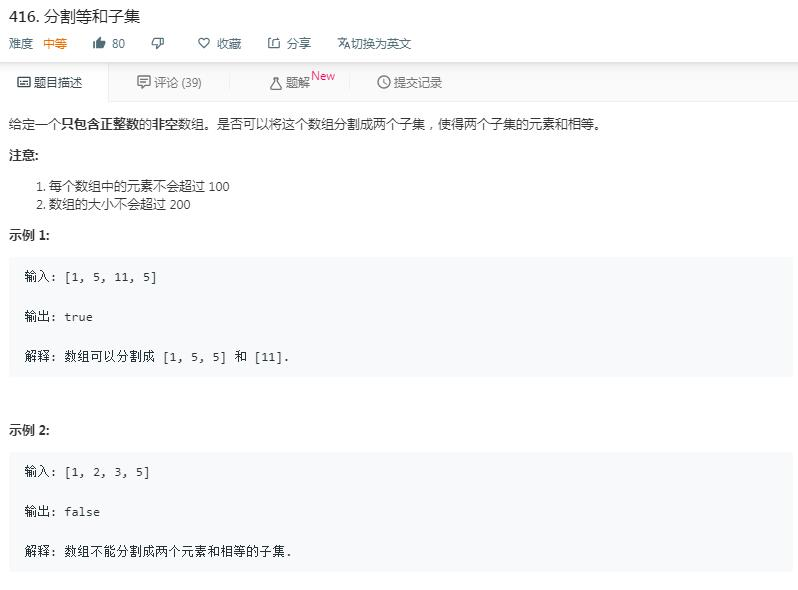是一个典型的背包问题，从某地方抽取元素，填充某个值

设sum为非空集合所有元素的和，若sum%2==0且其中有部分元素的和等于sum/2，
则剩下元素和必定为sum/2

抽象成背包问题则变成：从非空集合中，选出一定元素，填充sum/2的背包,如下图示：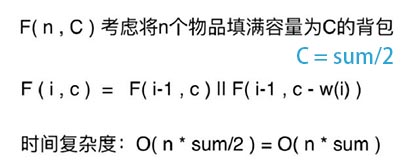func min(v1, v2 uint8) uint8 {
if v1 < v2 {
return v1
}
return v2
}

方法1:记忆化搜索
// 时间复杂度O(n^c)
// 空间复杂度O(n*c)
func canPartition(nums []int) bool {
sum := 0
n := len(nums)
for i := 0; i < n; i++ {
sum += nums[i]
}

if sum%2 != 0 {
return false
}

c := sum / 2

// 0代表未计算,1代表已计算且为true,2代表已计算且为false
memo := make([][]uint8, n)
for i := 0; i < n; i++ {
memo[i] = make([]uint8, c+1)
}

return tryPartion(nums, n-1, c, memo)
}

// [0...index]的数字中是否有能否刚好填充容积c的背包
func tryPartion(nums []int, index, c int, memo [][]uint8) (flag bool) {

if index < 0 || c < 0 {
//因为index和c都可能小于0,索引越界,这里只能直接返回,不能给memo赋值
return false
}

if memo[index][c] != 0 {
return memo[index][c] == 1
}

if c == 0 {
flag = true
} else {
// 2种情况,只要有1种成立即可
// 情况1: index位元素不放进c
flag = tryPartion(nums, index-1, c, memo) ||
// 情况1: index位元素放进c
tryPartion(nums, index-1, c-nums[index], memo)
}

// 存储计算结果
if flag {
memo[index][c] = 1
} else {
memo[index][c] = 2
}
return
}

方法2: 动态规划--二维数组
// 时间复杂度:O(n*c)
// 空间复杂度O(n*c)
func canPartition2(nums []int) bool {
n := len(nums)
if n < 2 {
return false
}

sum := 0
for i := 0; i < n; i++ {
sum += nums[i]
}

if sum%2 != 0 {
return false
}

c := sum / 2

// 0代表未计算,1代表已计算且为true,2代表已计算且为false
memo := make([][]uint8, n)
for i := 0; i < n; i++ {
memo[i] = make([]uint8, c+1)
}

// i行的情况:依赖i-1行求值
if nums > c {
return false
} else {
for i := 0; i <= c; i++ {
if i == nums {
memo[i] = 1
} else {
memo[i] = 2
}
}
}

// 第i行的值依靠i-2行,i行的值存在i-1行
for i := 1; i < n; i++ {
for j := c; j >= 0; j-- {
// 情况1
memo[i][j] = memo[i-1][j]
// 情况2
if j >= nums[i] {
memo[i][j] = min(memo[i][j], memo[i-1][j-nums[i]])
}
}
}
return memo[n-1][c] == 1
}

方法3: 动态规划--一维优化
// 时间复杂度:O(n*c)
// 空间复杂度O(c)
func canPartition3(nums []int) bool {
n := len(nums)
if n < 2 {
return false
}

sum := 0
for i := 0; i < n; i++ {
sum += nums[i]
}

if sum%2 != 0 {
return false
}

c := sum / 2

// true表示刚好填满,false无法刚好填满
memo := make([]bool, c+1)

//0行情况: nums>c,肯定不成立
if nums > c {
return false
} else {
memo[nums] = true
}

for i := 1; i < n; i++ {
for j := c; j >= nums[i]; j-- {
// 2种情况
// 1)不添加nums[i]能不能填满;2)添加nums[i]能不能填满
if memo[j] || memo[j-nums[i]] {
memo[j] = true
}
}
}
return memo[c]
}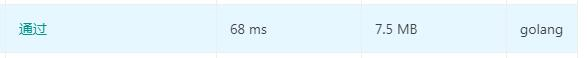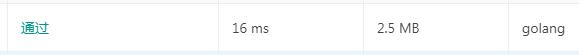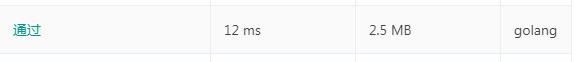1，2，3提交leetcode，通过


展开全文• 如何用动态规划求解呢? 核心思想就是：在当前背包所能容纳的容量下，装进某个物品的价值是多少，不装进这个物品的价值是多少 第一步： 初始化一个二维数组，定义为dp， 每一行表示的是，装进前i个物品时背包内的总...算法 python 数据结构
• 动态规划求解背包问题中的应用背包问题向来是动态规划的典型问题，给定n个重量为w1,w2,...,wn，价值为v1,v2,...,vn的物品和一个称重量为W的背包，求这些物品中最优价值的一个子集，且能够装到背包中。之前用蛮力法...DP java
• 今天下午又把 0-1 背包问题看了下，发现之前的写法虽然答案正确，但是和动态规划思想相关度不大。不是想当然地从一个二维数组的  元素开始求解。 直接放上代码把，因为已经写得很详细了。 /** 最优子结构：...算法
• 什么是动态规划动态规划是一种在数学和计算机科学中...比较著名的应用实例有：求解最短路径问题，背包问题，项目管理，网络流优化等。 动态规划在查找有很多重叠子问题的情况的最优解时有效。它将问题重新组...
• 这篇文章把动态规划过程剖析的很好 ...动态规划算法通常用于求解具有某种最优性质的问题。在这类问题中，可能会有许多可行解。每一个解都对应于一个值，我们希望找到具有最优值的解。动态规划算法与分治法类似，其算法
• 理论部分来自 ... 一、基本概念 ...一个决策序列就是在变化的状态中产生出来的，所以，这种多阶段最优化决策解决问题的过程就称为动态规划。 二、基本思想与策略  基本思想与分治法类似，也是将待求
• 动态规划的基本思想： 将一个问题分解为子问题递归求解，且将中间结果保存以避免重复计算。通常用来求最优解，且最优解的局部也是最优的。求解过程产生多个决策序列，下一步总是依赖上一步的结果，自底向上的求解...
• 0- 1 背包问题如果采用递归算法来描述则非常清楚明白, 它的算法根本思想是假设用布尔函数knap( s, n) 表示n 件物品放入可容质量为s 的背包中是否有解( 当knap 函数的值为真时 说明问题有解,其值为假时无解) . 我们...
• 动态规划法是将待求解问题分成若干个子问题，先求解问题，然后从这些子问题的解得到原问题的解。与分治法不同的是，适合用动态规划求解问题，经过分解的子问题往往不是独立的。若用分治法求解这类问题，则...
• 动态规划(Dynamic Programming，DP)，在平时的应用非常广泛，几乎在所有的比赛和面试当中都可以看到DP的身影，所以我来说一下什么是DP动态规划，然后顺便讲解一个非常经典的DP问题–背包问题。 DP基本思想 是一种在...
• 1.动态规划基本思想： （1）将原始问题划分一系列子问题； （2）求解每个子问题仅一次，并将其结果保存在一个表中，以后用到时直接存取，不重 复计算，节省计算时间； （3）自底向上计算。 2.解题四个部分： （1）...
• 算法之动态规划（DP）求解01背包问题 上面这篇文章主要讲解了01背包问题动态规划算法，如果你不了解动态规划算法，建议先浏览一下这篇文章熟悉一下，因为，本文的算法思想是基于这篇文章的。 1、什么是完全背包...动态规划 算法
• 动态规划 动态规划 Dynamic Programming，核心思想就是将大问题划分成小问题...01背包问题是经典的利用动态规划算法求解的一个经典案例 有n个物品，他们有各自的重量(w)和价值§，有一个给定容量capacity容量的背包，
• 动态规划算法介绍: 1.动态规划(Dynamic Programming)算法的核心思想是将大问题划分为小...3.和分治算法不同的是,用动态规划求解问题，分解的子问题往往不是独立的（下一个阶段的求解是建立在上一个阶段的基础上）...
• 3）与分治法不同的是，适合于用动态规划求解问题，经分解得到子问题往往不是互相独立的。（即下一个子阶段的求解是建立在上一个子阶段的解的基础上，进行进一步的求解） 4）动态规划可以通过填表的方式来逐步推进...
• ## 动态规划解决背包问题

千次阅读 热门讨论 2015-10-18 21:45:33
动态规划法的思想类似于分治法，基本思路就是把一个问题分解成若干个子问题求解问题，然后从子问题中得出原问题的解。因为用分治法解决问题，相同的子问题会被多次求解，所以如果将原来的解保存，就可以减少...算法
• 动态规划背后的基本思想非常简单。大致上，若要解一个给定问题，我们需要解其不同部分（即子问题），再合并子问题的解以得出原问题的解。 通常许多子问题非常相似，为此动态规划法试图仅仅解决每个子问题一次，从而...
• 目录动态规划介绍背包问题解决思路总结规律代码实现 动态规划介绍 动态规划(Dynamic Programming)算法的核心思想是：将大...与分治法不同的是，适合于用动态规划求解的问题，经分解得到子问题往往不是互相独立...算法 python
• 动态规划 动态规划 Dynamic Programming，核心思想就是将大问题划分成小问题...01背包问题是经典的利用动态规划算法求解的一个经典案例 有n个物品，他们有各自的重量(w)和价值§，有一个给定容量capacity容量的背包，...